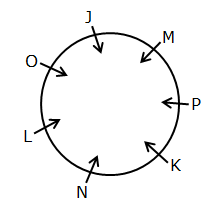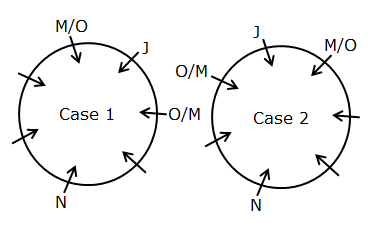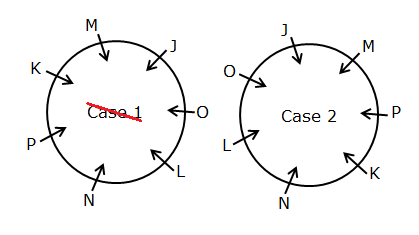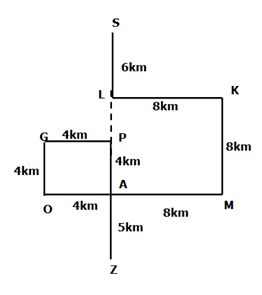# IBPS Clerk Prelims Reasoning Ability Questions 2021 (Day-04)

Dear Aspirants, Our IBPS Guide team is providing new series of Reasoning Questions for IBPS Clerk Prelims 2021 so the aspirants can practice it on a daily basis. These questions are framed by our skilled experts after understanding your needs thoroughly. Aspirants can practice these new series questions daily to familiarize with the exact exam pattern and make your preparation effective.

Start Quiz

Circular arrangement

Direction (1-5): Answer the questions based on the information given below.

Seven persons viz. J, K, L, M, N, O, and P are standing on a circular path such that all of them are facing towards the center, but not necessarily in the same order. The consecutive alphabetically named person doesn’t stand adjacent to each other. N stands three persons away from J. Only one person stands between M and O. P stands to the immediate right of K but not adjacent to N.

1) Who among the following person stands second to the right of K?

A.P

B.N

C.M

D.J

E.None of these

2) What is the position of L with respect to M?

A.Second to the right

B.Second to the left

C.Immediate left

D.Third to the right

E.Third to the left

3) How many persons are standing between J and P when counter from the left of J?

A.As many persons from the right of L to K

B.Three

C.As many persons from the left of J to M

D.Four

E.None of these

4) If all the persons are made to stand in reverse alphabetical order in a clockwise direction with respect to P, then how many persons remain unchanged in their position except P?

A.None

B.One

C.Two

D.Three

E.More than three

5) Who among the following person stands to the immediate left of the one who is third to the right of N?

A.M

B.J

C.O

D.P

E.None of these

Direction sense

Direction (6-8): Study the following information carefully and answer the below questions

Point K is 8km east of point L and 8km north of Point M. Point A is west of point M and South of point L. Point O is 4km west of point A and 4km south of point G which is west of point P. Point P is north of point A. Point Z is 5km south of point A. Point S is 6km north of Point L.

6) What is the direction of point O with respect to point L?

A.West

B.North-West

C.North-East

D.South-West

E.South-east

7) What is the shortest distance of point S with respect to point Z?

A.18km

B.20km

C.15km

D.19km

E.None of the above

8) Find the odd one out?

A.SK

B.ZO

C.PM

D.GA

E.GZ

Inequality

Direction (9-10): In each of the following questions, the relationship between different elements is shown in the statements followed by two conclusions. Find the conclusion which is definitely true.

a) If the Only conclusion I follow.

b) If Only conclusion II follows.

c) If either conclusion I or II follows.

d) If Neither conclusion I nor II follows.

e) If both conclusions I and II follow.

9) Statements:

B > D ≥ C = E < G ≤ F < H

Conclusions:

I). F > C

II). H < C

10) Statements:

P > S < W ≤ Q > M ≥ U

Conclusions:

I). S < Q

II). U < Q

Directions (1-5) :1) N stands three persons away from J. Only one person stands between M and O.The consecutive alphabetically named person doesn’t stand adjacent to each other.2) P stands to the immediate right of K but not adjacent to N. Hence, case 1 gets eliminated.Directions (6-8) :I). F > C (B > D ≥ C = E < G ≤ F < H) True

II). H < C (B > D ≥ C = E < G ≤ F < H) False